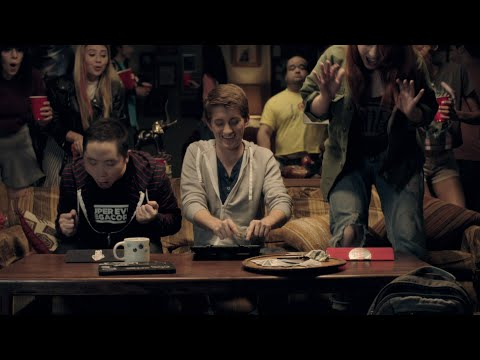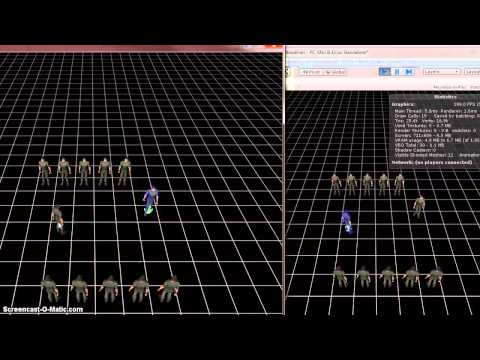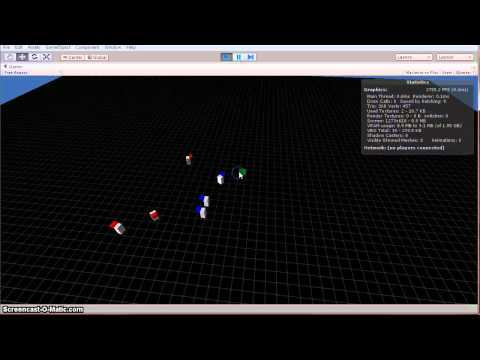# Finding linked faces within a 3d model with Unity C#

Recently I’ve been developing a global environment material shader. One of the main features of the shader is that it takes in relevant data through vertex information, so that all meshes can share the same material (in Unity3d C#). I thought it would be convenient to be able to tag mesh data inside the Unity editor per vertex, face or mesh within a mesh for multi-material models.

For something as useful as selecting meshes within a mesh, also known as elements in 3ds max or linked faces in Blender, there isn’t much information online about how to select them in code. So about a half day of thinking, coding and trial and error, I thought I’d post this solution for others seeking to implement the algorithm.

Problem: How do you get all the vertices of each unique surface within a mesh?
Solution: Given a single triangle face i of vertices, find all vertices of other faces containing i. Then run the same algorithm for each of the vertices found and output the list of all found vertices at the end of recursion.

I made a monobehaviour class called SetVertexColors. This class is assigned to the object which is meant to be edited.

First, a helper function to identify whether two faces are connected.

```        /// <summary>
/// Given two faces, return whether they share any common vertices.
/// </summary>

/// <param name="faceA">Face represented as array of vertex indices.</param>
/// <param name="faceB">Face represented as array of vertex indices.</param>
/// <returns>bool - whether the faces are connected. </returns>
bool IsConnected(int[] faceA, int[] faceB)
{
for (int i = 0; i < faceA.Length; i++)
for (int j = 0; j < faceB.Length; j++)
if (faceA[i] == faceB[j])
return true;
return false;
}
```

The magic happens in the below recursive function. It finds each linked face to the first face by iterating the index buffer, then recursively runs the same algorithm adding to the result.

My first instinct was to use a Hashset for the result so that there would not be duplicate values, though I thought List would be ok here since in Unity the vertex buffer is only 16 bits large, and it is the easiest container type to work with. Using a Hashset would have also resulted in another copy at the end for returning an array.

Also by using List in Unity with Mesh.GetTriangles() and managing it yourself, you can avoid copying the triangles buffer to a new array every time it is queried.

```        /// <summary>
/// Given a single triangle face of vertex indices,
/// returns a list of all the vertices of all linked faces.
/// </summary>

/// <param name="pickedTriangle">The known triangle to find linked faces from.</param>
/// <param name="triangles">The index buffer triangle list of all vertices in the mesh.</param>
/// <returns>List<int> - List of all the triangle face indices that represent the surface element.</returns>
public List<int> GetElement(int[] pickedTriangle, List<int> triangles)
{
// Create the return result list, starting with the current picked face
List<int> result = new List<int>(pickedTriangle);

// Iterate through the triangle list index buffer by triangle (iterations of 3)
for (int i = 0; i < triangles.Count; i += 3)
{
// Select the (i)th triangle in the index buffer
int[] curTriangle = new int { triangles[i], triangles[i + 1], triangles[i + 2] };

// Check if faces are linked
if (IsConnected(curTriangle, pickedTriangle))
{
// Recursively add all the linked faces to the result
}
}

return result;
}
```

For anyone interested that is also using Unity3d, here is my Editor script code for selecting a face in the scene view to pass to this function. The ray to face intersection triangle picking code is based on the Möller–Trumbore intersection algorithm, which is available here on Wikipedia.

```using System.Collections.Generic;
using UnityEngine;
using UnityEditor;

namespace Cyberdeck.Graphics
{
[CustomEditor(typeof(SetVertexColors))]
public class SetVertexColorsEditor : Editor
{
List<List<int>> m_triangles;
List<Vector3> m_vertices;

public void OnSceneGUI()
{

if (Event.current.type != EventType.MouseDown)
return;

if (Event.current.button != 0)
return;

SetVertexColors _target = (SetVertexColors)target;
Ray ray = HandleUtility.GUIPointToWorldRay(Event.current.mousePosition);
MeshFilter mf = _target.GetComponent(typeof(MeshFilter)) as MeshFilter;
Mesh mesh = mf.sharedMesh;

// Initialize temp buffer data if it doesn't exist
if (m_triangles == null)
{
m_triangles = new List<List<int>>(mesh.vertexCount / 3);
for (int i = 0; i < mesh.subMeshCount; i++)
}
if (m_vertices == null)
m_vertices = new List<Vector3>(mesh.vertexCount);

// Retrieve vertex positions from mesh. Using List prevents subsequent copying
mesh.GetVertices(m_vertices);

// Accumulate all of the faces an infinite ray may penetrate.
List<Vector4> candidates = new List<Vector4>();
for (int i = 0; i < mesh.subMeshCount; i++)
{
List<int> triangles = m_triangles[i];
mesh.GetTriangles(triangles, i);

for (int j = 0; j < triangles.Count; j += 3)
{
Vector3 p1 = m_vertices[triangles[j]];
Vector3 p2 = m_vertices[triangles[j + 1]];
Vector3 p3 = m_vertices[triangles[j + 2]];
p1 = _target.transform.TransformPoint(p1);
p2 = _target.transform.TransformPoint(p2);
p3 = _target.transform.TransformPoint(p3);
float dist = Intersect(p1, p2, p3, ray.origin, Vector3.Normalize(ray.direction));

if (dist >= 0)
candidates.Add(new Vector4(triangles[j], triangles[j + 1], triangles[j + 2], dist));
}
}

// If no intersections occured, user did not click on the mesh
if (candidates.Count == 0)
return;

// Sort the candidate faces for the closest one, which is the intuitivey picked face
Vector4 best = new Vector4(0, 0, 0, float.MaxValue);
for (int j = 0; j < candidates.Count; j++)
if (candidates[j].w < best.w)
best = candidates[j];

int[] pickedFace = new int[] { (int)best.x, (int)best.y, (int)best.z };
List<int> element = _target.GetElement(pickedFace, new List<int>(mesh.triangles), false);

// Calls the GetElement function, as well as setting vertex colors to the picked element.
_target.Apply(element);

Event.current.Use();
}

/// <summary>
/// Checks if the specified ray hits the triagnlge descibed by p1, p2 and p3.
/// Möller–Trumbore ray-triangle intersection algorithm implementation.
/// </summary>

/// <param name="V1">Vertex 1 of the triangle.</param>
/// <param name="V2">Vertex 2 of the triangle.</param>
/// <param name="V3">Vertex 3 of the triangle.</param>
/// <param name="ray">The ray to test hit for.</param>
/// <returns><c>true</c> when the ray hits the triangle, otherwise <c>false</c></returns>
public static float Intersect(Vector3 V1, Vector3 V2, Vector3 V3, Vector3 O, Vector3 D, bool backfaceCulling = true)
{
Vector3 e1, e2; //Edge1, Edge2
Vector3 P, Q, T;
float det, inv_det;
float t, u, v;

//Find vectors for two edges sharing V1
e1 = V2 - V1;
e2 = V3 - V1;

//Begin calculating determinant - also used to calculate u parameter
P = Vector3.Cross(D, e2);

//if determinant is near zero, ray lies in plane of triangle or ray is parallel to plane of triangle
det = Vector3.Dot(e1, P);

//if determinant is near zero, ray lies in plane of triangle otherwise not
// if the determinant is negative the triangle is backfacing
// if the determinant is close to 0, the ray misses the triangle
if (backfaceCulling)
if (det < Mathf.Epsilon) return -1f;
else
if (Mathf.Abs(det) < Mathf.Epsilon) return -1f;

inv_det = 1.0f / det;

//calculate distance from V1 to ray origin
T = O - V1;

//Calculate u parameter and test bound
u = Vector3.Dot(T, P) * inv_det;

//The intersection lies outside of the triangle
if (u < 0f || u > 1f) return -1f;

//Prepare to test v parameter
Q = Vector3.Cross(T, e1);

//Calculate V parameter and test bound
v = Vector3.Dot(D, Q) * inv_det;

//The intersection lies outside of the triangle
if (v < 0f || u + v > 1f) return -1f;

t = Vector3.Dot(e2, Q) * inv_det;
return t;
}

}
}
```

In this sample, I changed vertex colors on the mesh beyond just picking. Here is the full class to allow that functionality paired with the editor script.

```namespace Cyberdeck.Graphics
{
[ExecuteInEditMode()]
public class SetVertexColors : MonoBehaviour
{
public Color32 m_color;
private Color32 m_prevColor;
private List<Color32> m_colorsOut;
private Mesh m_mesh;
private List<List<int>> m_triangles;

void Initialize()
{
if (m_mesh == null)
m_mesh = GetComponent<MeshFilter>().sharedMesh;

if (m_triangles == null || m_triangles.Count != m_mesh.subMeshCount)
{
m_triangles = new List<List<int>>(m_mesh.subMeshCount);
for (int i = 0; i < m_mesh.subMeshCount; i++)
{
m_mesh.GetTriangles(m_triangles[i], i);
}
}

if (m_colorsOut == null)
{
m_colorsOut = new List<Color32>(new Color32[m_mesh.vertexCount]);
m_mesh.GetColors(m_colorsOut);
}

if (m_colorsOut.Count != m_mesh.vertexCount)
{
m_colorsOut = new List<Color32>(new Color32[m_mesh.vertexCount]);
}
}

public void Apply(List<int> element)
{
Initialize();

m_prevColor = m_color;

// Only set the colors for the selected element indices
for (int i = 0; i < element.Count; i++)
m_colorsOut[element[i]] = m_color;
m_mesh.SetColors(m_colorsOut);
}

/// <summary>
/// Given a single triangle face of vertex indices,
/// returns a list of all the vertices of all linked faces.
/// </summary>
/// <param name="pickedTriangle">The known triangle to find linked faces from.</param>
/// <param name="triangles">The index buffer triangle list of all vertices in the mesh.</param>
/// <param name="isDestructive"></param>
/// <returns></returns>
public List<int> GetElement(int[] pickedTriangle, List<int> triangles, bool isDestructive = true)
{
// Create the return result list, starting with the current picked face
List<int> result = new List<int>(pickedTriangle);

// Iterate through the triangle list index buffer by triangle (iterations of 3)
for (int i = 0; i < triangles.Count; i += 3)
{
// Select the (i)th triangle in the index buffer
int[] curTriangle = new int { triangles[i], triangles[i + 1], triangles[i + 2] };

// Check if faces are linked
if (IsConnected(curTriangle, pickedTriangle))
{
if (isDestructive)
{
triangles[i] = -1;
triangles[i + 1] = -1;
triangles[i + 2] = -1;
}

// Recursively add all the linked faces to the result
}
}

return result;
}

/// <summary>
/// Given two faces, return whether they share any common vertices.
/// </summary>
/// <param name="faceA">Face represented as array of vertex indices.</param>
/// <param name="faceB">Face represented as array of vertex indices.</param>
/// <returns>bool - whether the faces are connected. </returns>
bool IsConnected(int[] faceA, int[] faceB)
{
for (int i = 0; i < faceA.Length; i++)
for (int j = 0; j < faceB.Length; j++)
if (faceA[i] == faceB[j])
return true;
return false;
}

}
}
```

# Easy to manage event system in C# and Unity3d

.NET has a really nice event callback system that works great with Unity3d. This is important for a few reasons:

• Performance: Checking and running code through Update on every game object every frame can bogg down the application cpu thread.
• Organization: Using component based design, it is best to keep each component self contained. It can be easy to get in a tangled mess adding references all over different game objects to work together.
• Disabled Objects: Unity doesn’t run code on disabled objects and has strange behavior when trying to use a standard Constructor. This method works on disabled objects, allowing you to enable them on an event for example.

This is the event handler class:

```public class GameEvent : MonoBehaviour
{
// Add events to this enum as needed
public enum Type
{
GameStart,
GameEnd,
Win,
};

private GameEventListener[] m_listeners = null;
public delegate void Handler(Type e);
public static event Handler Callback;

// Collect all listeners on startup
void Awake()
{
m_listeners = Resources.FindObjectsOfTypeAll&lt;GameEventListener&gt;();

foreach (GameEventListener listener in m_listeners)
}

// Cleanup all listeners on shutdown
void OnDestroy()
{
foreach (GameEventListener listener in m_listeners)
listener.RemoveCallback(ref Callback);
}

// Call this to trigger events
public static void Trigger(Type e)
{
Callback(e);
}
}

To issue events, just call GameEvent.Trigger with the event type as the parameter. Then on game objects that need to listen and respond to events:
public abstract class GameEventListener : MonoBehaviour
{
public void AddCallback(ref GameEvent.Handler callback)
{
callback += new GameEvent.Handler(OnGameEvent);
}

public void RemoveCallback(ref GameEvent.Handler callback)
{
callback -= new GameEvent.Handler(OnGameEvent);
}

protected abstract void OnGameEvent(GameEvent.Type e);
}

Just extend from GameEventListener for your own classes to be able to listen and respond to events, like so:
class ExampleListener : GameEventListener
{
protected override void OnGameEvent(GameEvent.Type e)
{
if (e == GameEvent.Type.GameStart)
{
// Custom event response code here
}
}
}

The only drawback of this method is that objects that extend from GameEventListener cannot use Unity coroutines because of GameEventListener being abstract and messing with how Unity backend of Monobehavior works. This can be solved by using a thread pool. Or, if you need coroutines just implement the methods from GameEventListener in a class extended from MonoBehaviour. The reason GameEventListener is abstract is because extended objects would otherwise not be able to receive events when they are disabled because of how MonoBehavior prevents code running on disabled objects.
```

# Introducing VainGlory, as featured at the 2014 Apple keynoteVainGlory, my current project, is the awesome 3v3 MOBA perfected for touch devices. Of my own opinion, I joined this project because playing with touch on an iPad felt way nicer than playing a MOBA on PC, its just more elegant. Also the game is super fun.

Check out the game site www.vainglorygame.com!

Stephan and Tommy just showed the game at the Apple keynote.

And… the official VainGlory (Lan) Party trailer:# Old Projects, Old Engines

I happened to come across this link to an old Torque Engine posting from an early side project prototype (Cyberpunk, 2008).

One interesting idea here is for dynamic path-finding without pre-generated navigation grids or meshes. The main influences here are robotics AI and the study of insect behavior. Through all the reading and life experience on the subject, I’m convinced that insect behaviors can be accurately programmed into AI bots. What really excites me about dynamic path-finding is a future application to robotics. I could have used a pre-generated A* approach, but since this is a personal project I took interest in the challenge of dynamic path-finding in an unfamiliar environment because I think its really cool that it could theoretically be used in real-time for real robots.

Here is a link to the old post:
https://www.garagegames.com/community/blogs/view/15881

# Dante Confections, Chocolate Truffles Assortment Gift Box

Since 1989 my parents have been producing these fine gourmet chocolate truffles for candy and chocolate stores throughout New England. Dante’s chocolate truffles are now available online at flat rate shipping to anywhere in the U.S.

Chocolate Truffles Gift Box AssortmentThe 12 chocolate truffles gift box from Dante Confections is perfect for any occasion, whether for yourself or as a gift. These beautiful handcrafted gourmet truffles are made to perfection with fine detail and decoration. They are made with the finest mix of chocolate and are of the highest quality and taste. We are sure anyone who likes chocolate will love this truffle gift box.

Flavors: Dark Chocolate, Irish Cream Liqueur, Amaretto Liqueur, Milk Chocolate, Cherry Liqueur, Grand Marnier Liqueur, Caramel, Peanut Butter, Champagne Liqueur, Cappuccino, Raspberry, White Chocolate.

See our review on ChocolateRatings.com

Buy our gourmet chocolate truffles here: www.danteconfection.com

# Unity Prototype Update 4

Now added multiplayer support using the Photon service for unity. This is a mostly client trusting networking model, where the Photon server keeps track of players while passing network traffic between them. Also threw in selection highlight for characters using a rim light shader, and a selection bobber with double-click to move.# Unity Prototype Update 2

Ok, a few days later and already lots of feature updates to this prototype.

– Grid movement system. Based off snapping coordinates to a X/Z grid
– Selection system, highlighter for valid and invalid moves. Valid moves are based off of raycast collision with a valid tagged asset. Invalid moves are if the cursor is too many grid tiles away, or if raycast collision with an invalid tagged asset.
– Sub-Unit character system, controls action points and movement for each character the player controls.
– Turn system, able to keep track of multiple players. Activates valid player and sub-units and switches active player when all sub-unit action points are spent.